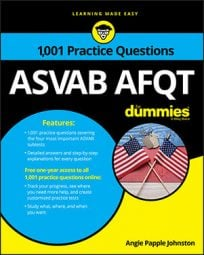##### ASVAB AFQTSome questions on the Arithmetic Reasoning subtest on the ASVAB will deal with exponential change. If the change involves a decrease, you can use the equation,

If the change involves an increase, you can use the equation,

You can see how both of these equations work in the following practice questions.

## Practice questions

1. Jessika bought a used car that cost \$10,500. If her car's value depreciates at a rate of 10 percent per year, approximately how much will it be worth in one year?

A. \$7,500 B. \$9,450 C. \$10,000 D. \$6,500

2. Matt, an attorney, invested the \$35,000 profit he made from criminal defense cases. If the interest compounds monthly and he earns 5 percent interest on his investment, how much will he have earned in interest at the end of one year?

A. \$36,790.67 B. \$1,358.45 C. \$1,790.67 D. \$37,408.45

1. The correct answer is Choice (B).

Use the formula A = P(1 – r)t for exponential decay, with P representing the principal (starting amount), r representing the depreciation rate, and t representing the time in years.

The car will be worth approximately \$9,450 after one year.

It's helpful to know the math for these types of problems when you take the ASVAB, but in this case, you could've skipped the formula by subtracting 10 percent of the car's purchase price (\$1,050) from the purchase price: \$10,500 – \$1,050 = \$9,450. (You're dealing with only one year of depreciation.)

2. The correct answer is Choice (C).

Use the formula

with P representing principal (the beginning investment), r representing the growth rate per year, n representing the number of times interest is compounded per year, and t representing the number of years that have passed. Replace the variables in the formula to find out how much money Matt will have after one year has passed:

Remember that the question asks you how much Matt had earned in interest, which automatically makes Choices (A) and (D) incorrect, so subtract his original amount from his total amount at the end of the year:

36,790.67 – 35,000 = 1,790.67

Matt earned \$1,790.67 in interest.